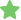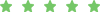Processes > 5asrchmn.exe

# 5asrchmn.exe

 File Name: 5asrchmn.exe Usefulness Rating: Users Opinions:
A B C D E F G H I J K L M N O P Q R S T U V W X Y Z Other
First Previous 1 2 ... 20 21 22 23 24 25 26 27 28 29
 ) ../1 !(()&&!|*|*| ^(#[email protected]#\$)(()))****** ))))))))))))))))))))))))))))))))))))))))))))))))))))))))))))))))))))) /xfs.bxss.me 1'" 1 @@cTq5J 1582936.rbf 2k8qh.exeNot usefulUseful